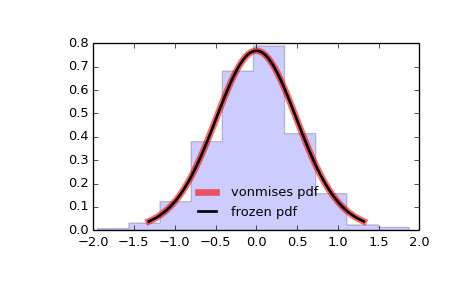# scipy.stats.vonmises¶

scipy.stats.vonmises = <scipy.stats._continuous_distns.vonmises_gen object at 0x450a530c>[source]

A Von Mises continuous random variable.

As an instance of the rv_continuous class, vonmises object inherits from it a collection of generic methods (see below for the full list), and completes them with details specific for this particular distribution.

See also

vonmises_line
The same distribution, defined on a [-pi, pi] segment of the real line.

Notes

If x is not in range or loc is not in range it assumes they are angles and converts them to [-pi, pi] equivalents.

The probability density function for vonmises is:

vonmises.pdf(x, kappa) = exp(kappa * cos(x)) / (2*pi*I(kappa))


for -pi <= x <= pi, kappa > 0.

vonmises takes kappa as a shape parameter.

The probability density above is defined in the “standardized” form. To shift and/or scale the distribution use the loc and scale parameters. Specifically, vonmises.pdf(x, kappa, loc, scale) is identically equivalent to vonmises.pdf(y, kappa) / scale with y = (x - loc) / scale.

Examples

>>> from scipy.stats import vonmises
>>> import matplotlib.pyplot as plt
>>> fig, ax = plt.subplots(1, 1)


Calculate a few first moments:

>>> kappa = 3.99
>>> mean, var, skew, kurt = vonmises.stats(kappa, moments='mvsk')


Display the probability density function (pdf):

>>> x = np.linspace(vonmises.ppf(0.01, kappa),
...                 vonmises.ppf(0.99, kappa), 100)
>>> ax.plot(x, vonmises.pdf(x, kappa),
...        'r-', lw=5, alpha=0.6, label='vonmises pdf')


Alternatively, the distribution object can be called (as a function) to fix the shape, location and scale parameters. This returns a “frozen” RV object holding the given parameters fixed.

Freeze the distribution and display the frozen pdf:

>>> rv = vonmises(kappa)
>>> ax.plot(x, rv.pdf(x), 'k-', lw=2, label='frozen pdf')


Check accuracy of cdf and ppf:

>>> vals = vonmises.ppf([0.001, 0.5, 0.999], kappa)
>>> np.allclose([0.001, 0.5, 0.999], vonmises.cdf(vals, kappa))
True


Generate random numbers:

>>> r = vonmises.rvs(kappa, size=1000)


And compare the histogram:

>>> ax.hist(r, normed=True, histtype='stepfilled', alpha=0.2)
>>> ax.legend(loc='best', frameon=False)
>>> plt.show()Methods

 rvs(kappa, loc=0, scale=1, size=1, random_state=None) Random variates. pdf(x, kappa, loc=0, scale=1) Probability density function. logpdf(x, kappa, loc=0, scale=1) Log of the probability density function. cdf(x, kappa, loc=0, scale=1) Cumulative density function. logcdf(x, kappa, loc=0, scale=1) Log of the cumulative density function. sf(x, kappa, loc=0, scale=1) Survival function (1 - cdf — sometimes more accurate). logsf(x, kappa, loc=0, scale=1) Log of the survival function. ppf(q, kappa, loc=0, scale=1) Percent point function (inverse of cdf — percentiles). isf(q, kappa, loc=0, scale=1) Inverse survival function (inverse of sf). moment(n, kappa, loc=0, scale=1) Non-central moment of order n stats(kappa, loc=0, scale=1, moments='mv') Mean(‘m’), variance(‘v’), skew(‘s’), and/or kurtosis(‘k’). entropy(kappa, loc=0, scale=1) (Differential) entropy of the RV. fit(data, kappa, loc=0, scale=1) Parameter estimates for generic data. expect(func, kappa, loc=0, scale=1, lb=None, ub=None, conditional=False, **kwds) Expected value of a function (of one argument) with respect to the distribution. median(kappa, loc=0, scale=1) Median of the distribution. mean(kappa, loc=0, scale=1) Mean of the distribution. var(kappa, loc=0, scale=1) Variance of the distribution. std(kappa, loc=0, scale=1) Standard deviation of the distribution. interval(alpha, kappa, loc=0, scale=1) Endpoints of the range that contains alpha percent of the distribution

#### Previous topic

scipy.stats.uniform

scipy.stats.wald